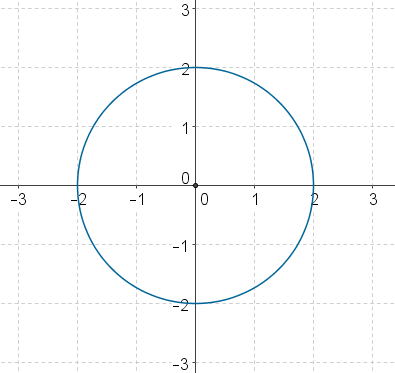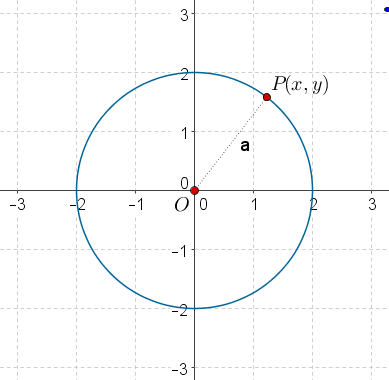# Circles Centered at the Origin

Go back to  'Coordinate-Geometry'

The following figure shows a circle of radius 2 units centered at the origin:What will be the equation of this circle? In other words, what will be that relation which every point on this circle satisfies, but no point not on this circle satisfies?

Let $$P\left( {x,y} \right)$$ be an arbitrary point lying on this circle. What is the constraint on P?The constraint is that the distance of P from the origin (OP) must be 2 units. Using the distance formula, we have:

$\begin{array}{l}OP = 2\\ \Rightarrow \,\,\,\sqrt {{{\left( {x - 0} \right)}^2} + {{\left( {y - 0} \right)}^2}} = 2\\ \Rightarrow \,\,\,\sqrt {{x^2} + {y^2}} = 2\\ \Rightarrow \,\,\,{x^2} + {y^2} = 4\end{array}$

This is the equation that the coordinates of every point lying on the circle will satisfy (and no point not lying on the circle will satisfy). Thus, we can say that this is the equation of the circle.

In general, for a circle of radius r centered at the origin, its equation will be

${x^2} + {y^2} = {r^2}$

Example 1: What is the radius of the circle whose equation is $${x^2} + {y^2} = 20$$?

Solution: Comparing this with the equation $${x^2} + {y^2} = {r^2}$$, we see that

${r^2} = 20\,\,\, \Rightarrow \,\,\,r = \sqrt {20} = 2\sqrt 5$

Example 2: Consider the following two concentric circles:

$\begin{array}{l}{x^2} + {y^2} = 9\\{x^2} + {y^2} = 16\end{array}$

What is the area of the ring formed by these two circles?

Solution: The radius of the smaller circle is 3 units, and that of the larger circle is 4 units. Thus, the area of the ring enclosed between them will be

$\pi {\left( 4 \right)^2} - \pi {\left( 3 \right)^2} = 7\pi \,{\rm{sq}}{\rm{.units}}$

Coordinate Geometry
Grade 9 | Questions Set 1
Coordinate Geometry
Coordinate Geometry
Grade 10 | Questions Set 1
Coordinate Geometry